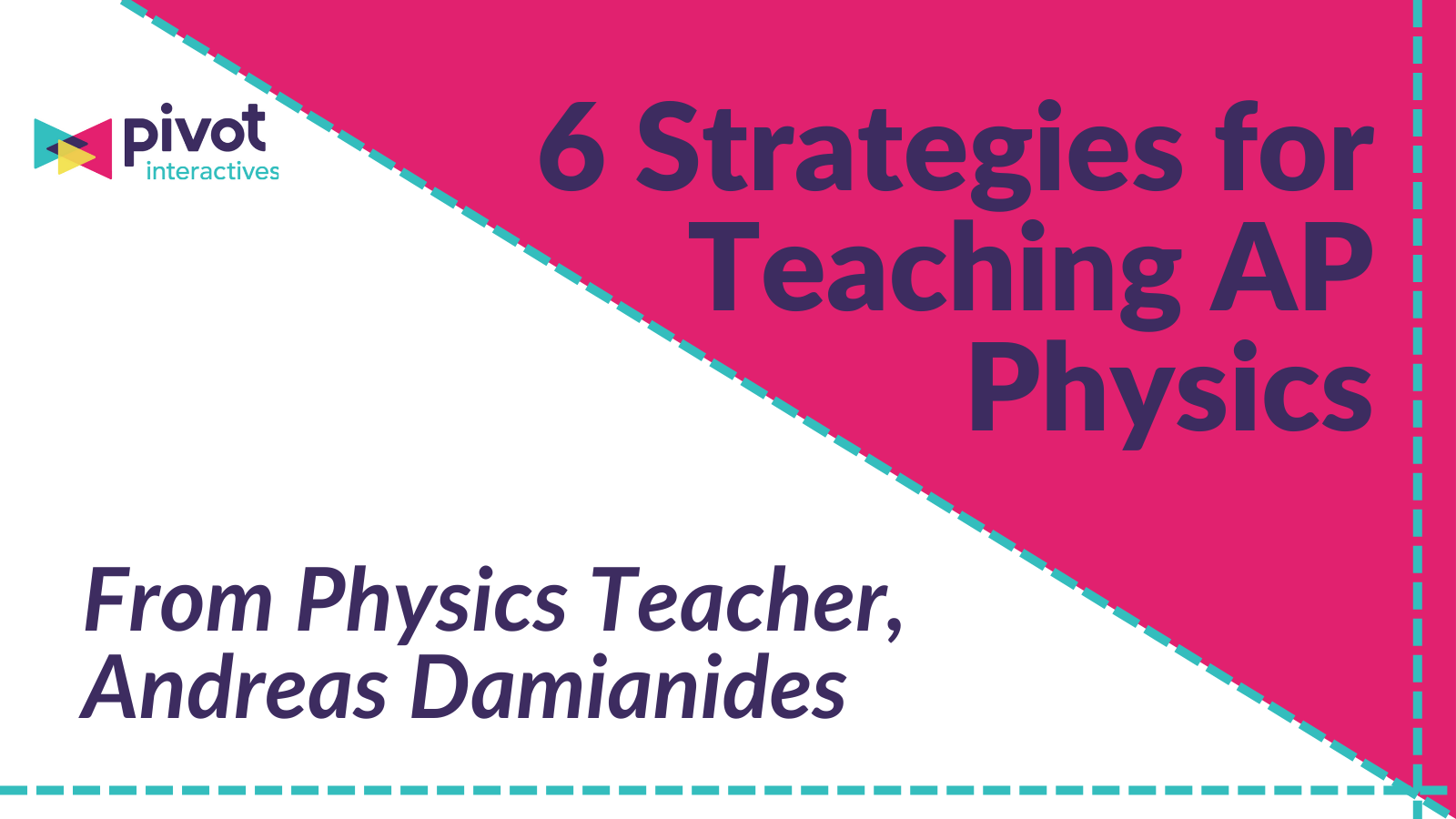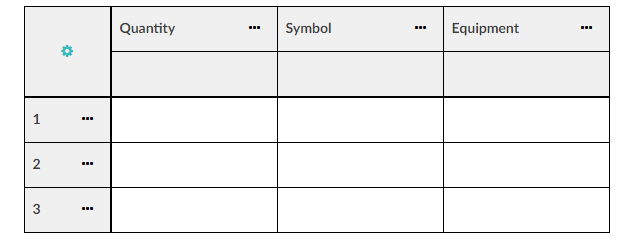# 6 Tips for Teaching AP Physics

August 9, 2022# 6 Tips for Teaching AP Physics

## Read the 6 strategies Andreas used to help his student better understand AP Physics, engage with the science practices using real phenomena, and increase his class AP Physics test pass rate.

#### Andreas Damianides is a high school Physics and AP Physics teacher at St. Charles CUSD 303 in Illinois. He also develops curriculum and assessments aligned to Next Generation Science Standards (NGSS).

Teaching AP Physics can be a daunting task, even for the most experienced teachers. As someone who has been teaching this class for close to a decade, I have found some useful approaches that have been particularly effective in increasing AP Physics scores, but more importantly have improved students' conceptual understanding of important physics concepts. These strategies took my class from a more equation and calculation based course to a course designed around understanding mathematical relationships and scientific concepts. After shifting my teaching approach, my class's pass rate improved by ~25% and is significantly above the global average.

## Here are 6 strategies that help facilitate that improvement:

### 1. Use the Same Examples Throughout Units

Many physics problems can be solved in multiple ways and in multiple  units within the course. It is extremely valuable for students to realize that as long as they use the right values in the right place, they can solve a problem using almost any unit. I found this strategy at an AP symposium about 5 years ago and have embraced it ever since.

One example of what this might look like is a problem involving a roller coaster sliding to a stop due to friction. I use this same scenario in the kinematic, dynamics, energy, and momentum units and solve the problem using graphs, equations, or both. If you are trying to find the value of d, you could use the area of a velocity time graph. If you want to find the time to stop, you can use impulse. If you want to find the coefficient of friction, you can use kinematics and dynamics or you can use work and dynamics. Using the same prompts to explain concepts helps students to understand the connections between the units in mechanics and that they can be used interchangeably and in tandem with each other.

### 2. Encourage Students to Make Meaning from Weird Graphs

One thing that students immediately struggle with is how to make meaning out of graphs. To help students make the connection between what is on the graph and the analytical factors, I will use the same data and graph different functions to help students make the connection.

For example, this Pivot Interactives activity that asks students to analyze the period of a harmonic oscillator and derive the spring constant. When my students complete this task, I have some students that graph period vs. √m, 4π^2m vs. T^2 , or any number of options. What’s most important is that students are able to connect the equation for the period of a spring to the expressions they have chosen to graph. In the first example, the slope of the graph will be a function that needs to be manipulated to find the spring constant, but the second example has graphed values that provide the spring constant exactly as the slope. Using phenomena and the graphing tools in Pivot Interactives, this helps them see that both strategies give the same value in different ways!

### 3. Provide Structure for Experimental Design

Inquiry of some type is highly emphasized in any science class, whether following  NGSS, NBCT, College Board, or other standards. However, to help students be successful, they often need some structure. One way to do this is to outline the steps they will need to take, mimicking the structure of experimental design questions on the AP exam:

- Identify what data you are going to analyze and what you will need to graph (I need to find the relationship between _____ and ____) Do not let them do anything until they have written that statement and identified at least 2 things they need to measure.

- Fill out the Table (this helps students recognize what they will need to measure, what variable will represent that value, what unit they will use, and what tool will measure it.) This table is a mainstay on the AP test, however, it is also an extremely useful organizational tool to reduce the cognitive load on students.Pivot Interactives makes a question like this easy for teachers and students. In addition to the phenomena-based interactive videos, there are easy-to-use tools for creating tables and graphs. I easily customize the activities to scaffold the design process for students.

- Students can then carefully explain how each variable listed will be measured using each tool they have chosen. Remind students that measurements are not calculations.

- Now students can discuss how those measurements are going to be used by incorporating any necessary calculations or manipulations to arrive at a conclusion.

### 4. Help Students Understand what Equations Actually Mean

Practice Proportional Reasoning: Proportional Reasoning is a means by which students analyze the relationship between variables and the scale at which those variables influence one another. This skill helps students save time on the AP test, but more importantly, it helps them with number sense and what equations actually mean. It also helps them recognize why some graphs might have a certain shape before they are linearized.

Here is how I teach students to approach proportional reasoning:

• Write out the equation that relates the two variables in question
• Replace the variables that are not changing with a 1
• Replace the variables that are changing with the factor they are changing by

Let’s look at an example using Universal Gravitation:

In these examples, you can see how proportional reasoning helps students bypass the tedious calculations associated with Universal Gravitation and instead focus on how each variable affects the Force in question.

### 5. Practice What If’s

This style of question pops up all the time on the AP FRQs but is also just a fun exercise in algebraic manipulation. Essentially, you help students practice the skill of identifying how variables relate to each other. It is another example of a time when consistent prompts/examples can come in handy to help students see connections across variables, units, and topics.

Let’s go back to the roller coaster stopping distance problem:

You can quickly have students generate equations for minimum coefficients of friction at different velocities, starting heights to stop at certain distances or any number of options. This part of the class is called “Math without Numbers” and becomes an exercise in algebraic manipulation.

For example: you have generated an example equation using energy for stopping distance given an initial velocity v. You would have an equation that related Initial Kinetic Energy, GPE, and Work done by friction: 1/2mv^2=mgh + Fd.

To help students with their algebraic manipulation skills, you can then have them rearrange the equation to solve for any variable as practice.

• Solving for stopping distance becomes d=(1/2mv^2-mgh)/F.
• The “What if” part comes next. You can now ask students hypothetical questions regarding a change in a different variable and how it will impact the stopping distance. The derived equation helps them find this relationship and I tell them it is as simple as whether a variable is on the top or the bottom many times.
• If we increase velocity, the car will be going faster so it will be harder for it to slow down. Our equation backs that up because velocity is on the top of the equation meaning an increase in v will cause an increase in d
• If we increase the Force of friction, our stopping distance decreases because there is more force slowing it down so it doesn't have to travel as far to stop. The equation supports this because Force is in the denominator meaning an increase in F would cause a decrease in d

Connecting the logical reasoning component to the equation helps students connect the math to their actual experiences.

### 6. Engage Students in DOING

“There is no amount of Youtube dunk compilations that I can watch that will make me be able to dunk a basketball.”

At the end of the day, students can only get so much out of watching a tutorial video or reading a textbook. Students need to be engaged in the learning process in order to effectively learn. I like to tell my students, “there is no amount of Youtube dunk compilations that I can watch that will make me be able to dunk a basketball.” The tools provided by Pivot Interactives allow students to do things like generate their own graphs and gather their own data.

You can start with simple data and graphs like in the 1-D Kinematics Billiard Race. This activity helps students practice their graphing skills by looking at y-intercepts and slopes and relating them to one-dimensional motion. I love to use Pivot Interactives activities that are difficult to replicate in my own classroom setting, but provide engaging and fun contexts for physics problems. Activities like the Fire Extinguisher Rocket Cart enable students to investigate novel scenarios that apply physics concepts, like impulse, but are familiar to students.

It is critical that students consistently practice the content-specific skills required to learn physics, but they also need an avenue to practice the key science and engineering skills in unique and engaging ways; Pivot Interactives provides that in a way that is easy for teachers and valuable for students.

They also need an avenue to practice the key science and engineering skills in unique and engaging ways;

Pivot Interactives has been an invaluable supplement to my Physics & AP Physics classroom, giving me ways to engage students with the science practices through real phenomena often.

There are countless strategies to help students get ready for the AP Physics test, but these are some that have been most impactful to me. Find like-minded teachers, more ideas, & resources on the AP Physics Teachers Facebook page, using #iteachphysics on Twitter, or asking the Pivot Interactives team.  I hope you find these helpful and good luck this year!

To learn more about Pivot Interactives, try an activity for yourself, start a free trial, or send us a message by clicking the chat in the bottom right!

AP is a registered trademark by the College Board which is not affiliated with and does not endorse Pivot Interactives.